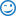Register Blogs Advertise with us Help Desk Today's Posts Search

This blog explores a newly-discovered passive plug-in that will double your Internet Marketing profits, and works on any WSO.

# Rule of 72: Are YOU making this mistake?Posted 8th July 2014 at 11:44 PM by David Beroff

You may have already heard of the "Rule of 72". It seems easy enough: To figure out how many years it will take an investment to double its value, just divide 72 by the interest rate.

So if you're earning 6% APY, it'll take 72 / 6 = 12 years to double. If an investment has a 8% yield, you're looking at 72 / 8 = 9 years.

OK, so what's the problem? This seems straight-forward; why am I even writing a blog post about this?

Here's the thing: The Rule of 72 is simply an approximation. It works pretty well at "traditional" rates of return. The rule gives an accurate result (within around 2%-3% of reality) for rates of return in the range of 2% to 12%, so finance people have relied on it for centuries. (Wikipedia actually shows a reference to the rule going back to 1494!)

"Traditional"... hrmm... what is your bank paying you on that "high yield" CD?The problem with the Rule of 72 is that it starts to break down a bit as you get involved with serious investments that pay you more than single-digit returns, like the investment club that I've been describing in this blog.

Let's say you are earning 2% per month instead of per year. That's 24% APR and 26.8% APY. The Rule of 72 would predict a doubling in a bit more than 32 months. The actual, correct answer is found by dividing natural logarithms, ln(2) / ln(1.268), which works out to 35 months. In other words, at that rate, you can double your money every three years. While the Rule of 72 did give a more attractive-looking answer, it was off by more than 9%. I'd hate for you to make such a large mistake.

So, as you can see, the right way to get an accurate answer to how long it'll take for an investment to double is to take ln(2) divided by ln(1+r). If your calculator only has ten-based logarithms, you can find the exact same ratio with log(2) / log(1+r).

If you have any questions, please message me, or simply email me: David (at) Beroff (dot) com.
_____
Full disclosure: Yes, of course I earn referral income when you join.
And you'll be able to do so, as well. :-) Past performance is never a
guarantee of future returns. Only invest what you can afford to lose.
Posted in investing